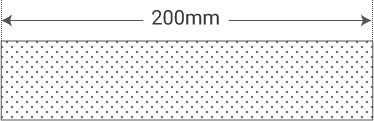Measurement Environment

# Assessing Measurements

Assessing measured values is no simple matter. A measured value is never a true value―some amount of error is always included. As such, it is difficult to assess the reliability of any one measurement. Conventionally, reliability was evaluated based on measured value bias (systematic error) and variations (random error) while using the true value as a reference.
However, the measured value assessments are not uniform, making it difficult to determine the true value. Therefore, a method for judging the reliability of the measurement results from a statistical perspective was devised. This is the concept of "uncertainty." The range in which the true value exists is calculated by using statistical processing to estimate the error.
For example, the length of a processed metal bar can be expressed as being measured as 200 mm long, with an uncertainty of ±0.01 mm and a level of confidence of 95%. This indicates that the true value is between 199.99 and 200.01 mm with a level of confidence of 95%.
This approach is used in international standards such as those of the International Organization for Standardization (ISO).Uncertainty: ±0.01 mm Level of confidence: 95%

INDEX